Lakhmir Singh & Manjit Kaur: Work and Energy, Solutions- 3

# Lakhmir Singh & Manjit Kaur: Work and Energy, Solutions- 3 - Science Class 9

Page 163
Solution 17
Force, F = 400 N
Distance, s = 60 m
Time taken, t = 1 minute = 60 s
Work done, W = F x s = 400 x 60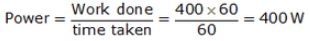Solution 18
At a hydroelectric power station, the potential energy of water is transformed into kinetic energy and then into electrical energy.
Solution 19
At a coal-based thermal power station, the chemical energy of coal is transformed into heat energy, whhich is further converted into kinetic energy and electrical energy.
Solution 20
Weight of the man = 500 N
Weight of the load = 100 N
Total weight = 600 N
Height of stairs = 4 m
Time taken = 5 s
Work done = mg x h = weight X h = 600 x 4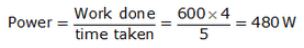Solution 21
Power = 3 kW
Time = 20 s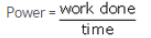Work done = power x time = 3 x 20 kWs = 60 kJ

Solution 22
Energy consumed = 600 kJ
Time taken = 5 minutes = 300 s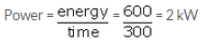Solution 23
Power = 100 W
a) time = 1 s
energy = power x time = 100 J
b) time = 1 minute = 60 s
energy = power x time= 100 x 60 = 6 kJ
Solution 24
Power of 1 fan = 120 W
Power of 5 fans = 5 x 120 = 600 W = 0.6 kW
Time = 4 hours
Electrical energy = 0.6 x 4 = 2.4 kWh
Solution 25
A radio first converts electrical energy into kinetic energy and then into sound energy
Solution 26
In an electric bulb, electrical energy is first converted into heat energy and then into light energy
Solution 27
Fan, washing machine, mixer grinder, water pump, hair dryer use electric motor
Solution 28
i) chemical energy to electrical energy
ii) electrical energy to heat and light energy
Solution 29
i) Maximum potential energy is present in the bob at point C as at point C bob is at maximum height.
ii) Maximum kinetic energy is present in the bob at point A as at point A bob is at maximum speed
Solution 30
Weight of the car = 20000 N= 20 kN
Speed = 8 m/s
Distance s= 120 m
Time = 100 s
a)Work done W = f x s = 20 x 120 = 2400 kJ

b) Power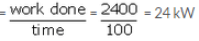Solution 31
a)The change of one form of energy into another form of energy is known as transformation of energy, e.g. in a cell chemical energy is transformed into
electrical energy
b) a. When a ball is thrown upwards its kinetic energy gradually converts into potential energy and potential energy
becomes maximum at the maximum height attained by the ball
b. When a stone is dropped from the roof of the building its potential energy gradually converts into kinetic energy and kinetic energy becomes maximum when the stone is just above the ground
Solution 32
a) Law of conservation of energy states that whenever energy changes from one form to another form, the total amount of energy remains constant. Energy can
never be created nor destroyed, it transforms from one form to another. For example, when electrical energy is converted into light energy in an electric
bulb, then some energy is wasted as heat during conversion but the total energy remains the same.
b) Initially the pendulum is at rest. The bob is pulled to one side to position B to give it potential energy due to higher position of B and then released, the bob
starts swinging
i. When the bob is at position B, it has potential energy but no kinetic energy
ii. As the bob starts moving down from position B to A, its potential energy starts decreasing and kinetic energy starts increasing
iii. When the bob is at position A, it has maximum kinetic energy and zero potential energy
iv. As the bob starts moving down from position A to C, its kinetic energy starts decreasing and potential energy starts increasing
v. On reaching the extreme position C, the bob stops for a very small instant of time and bob maximum potential energy and zero kinetic energy.
Therefore at extreme positions B and C bob has only potential energy and at A it has only kinetic energy and at other intermediate positions bob has both kinetic
and potential energy. Thus the total energy of the pendulum is same for any instant of time (conserved)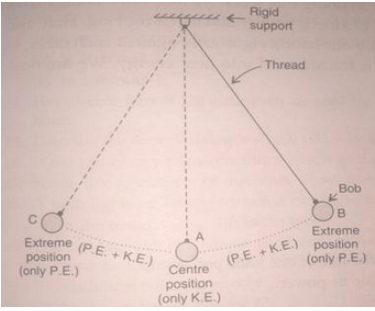Solution 33
a) The unit kWh stands for kilowatt-hour. One kilowatt-hour is the amount of electrical energy consumed when an electrical appliance having power of 1 kilowatt is used for 1 hour. It represents the amount of electrical energy consumed in 1 hour.
b) Power = 1000 W = 1 kW
Time = 60 minutes = 1 hour
Energy(kWh) = 1 x 1 = 1 kWh
Solution 34
a) 1 kilowatt-hour = 1 kW for 1 hour = 1000 W for 1 hour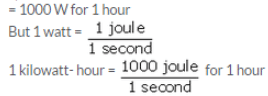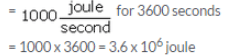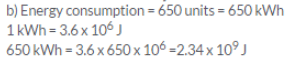Solution 35
a) Power is the rate of doing work.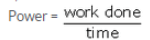Si unit of power is watt(w)

(b) Mass of the body = 40 kg

Mass of the box = 20 kg

total Mass = 60 kg

Height h = 15m

g= 10m/s2

time taken =25s

woek done = m x g x h = 60 x 15 x 10 = 9000 J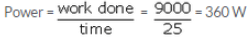Page 164
Solution 46
Mass of body = 1 kg
g = 10 m/s2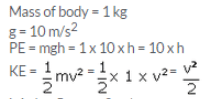I. At h= 5m; v= 0 m/s

PE = 10 x 5 = 50 J

KE = 0 J

at total Energy = PE+KE = 50 J

ii. at h = 3.2 m; v =10 m/s

PE = 0 J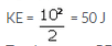total Energy = PE+KE = 50 J

The total energy in all three cases is constant . this prove the law of conservation of energy

Solution 47
Since from point A total energy = PE + KE = 80 J
And according to the law of conservation of energy Total energy remains constant
a) PE = 0
Total energy = PE + KE = 80 J
KE = 80 J PE = 80 J
b) At point B KE = 48 J
Total energy = PE + KE = 80 J
PE = 80 J KE = 80 – 48 = 32 J
c) Law of conservation of energy

Page 165
Solution 48
No. of steps = 28
Height of each step = 28 cm
Total height = 20 x 28 = 560 cm = 5.6 m
Mass of student = 55 kg
g = 9.8 m/s2
time = 5.4 s
Work done = m x g x h = 55 x 9.8 x 5.6 = 3018.4 J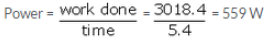Solution 49
Weight of box = 100 N
Height = 1.5 m
work done = m x g x h = 100 x 1.5 = 150 J
potential energy = m x g x h= 100 x 1.5 = 150 J
iii. weight of 4 boxes = 400 N
time = 1 minute = 60 s
work done = 400 x 1.5 = 600 J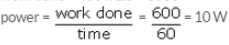Solution 50
a) Electrical energy to sound energy
b) Sound energy to electrical energy
c) Electrical energy to light (and heat) energy
d) Chemical energy to electrical energy to light
energy(and heat energy)

The document Lakhmir Singh & Manjit Kaur: Work and Energy, Solutions- 3 | Science Class 9 is a part of the Class 9 Course Science Class 9.
All you need of Class 9 at this link: Class 9

## FAQs on Lakhmir Singh & Manjit Kaur: Work and Energy, Solutions- 3 - Science Class 9

 1. What is work and energy?Ans. Work and energy are related concepts in physics. Work is defined as the transfer of energy that occurs when a force is applied to an object and the object is displaced in the direction of the force. Energy, on the other hand, is the ability to do work or cause change. It exists in different forms such as kinetic energy, potential energy, and thermal energy.
 2. How are work and energy related?Ans. Work and energy are closely related as work is the transfer of energy. When work is done on an object, energy is transferred to that object, causing a change in its state or motion. Similarly, when work is done by an object, it transfers its energy to another object or system. In simple terms, work is the process of transferring energy from one object to another.
 3. What are the different types of energy?Ans. There are several different types of energy. Some common forms of energy include: 1. Kinetic energy: This is the energy of motion. It depends on the mass and velocity of an object. For example, a moving car has kinetic energy. 2. Potential energy: This is the energy that an object possesses due to its position or condition. Examples include gravitational potential energy and elastic potential energy. 3. Thermal energy: This is the energy associated with the temperature of an object. It is related to the motion of particles within the object. 4. Chemical energy: This is the energy stored in chemical bonds. It is released or absorbed during chemical reactions. 5. Electrical energy: This is the energy associated with the flow of electric charge. It is used to power electrical devices and systems.
 4. How is work calculated?Ans. Work is calculated using the formula: Work = Force x Distance x cos(angle) where: - Force is the magnitude of the force applied to an object - Distance is the displacement of the object in the direction of the force - Angle is the angle between the force vector and the displacement vector The unit of work is joules (J). If the force and displacement are in the same direction, the angle will be 0 degrees and the cosine of 0 degrees is 1, resulting in the simplified formula: Work = Force x Distance
 5. What is the principle of conservation of energy?Ans. The principle of conservation of energy states that energy cannot be created or destroyed, but it can be transformed from one form to another or transferred between objects. In a closed system where no external forces are acting, the total amount of energy remains constant. This means that the total energy at the beginning of a process is equal to the total energy at the end, even if it changes forms.

## Science Class 9

98 videos|369 docs|148 tests

## Science Class 9

98 videos|369 docs|148 tests
Signup to see your scores go up within 7 days! Learn & Practice with 1000+ FREE Notes, Videos & Tests.
10M+ students study on EduRev
Track your progress, build streaks, highlight & save important lessons and more!(Scan QR code)
Related Searches

,

,

,

,

,

,

,

,

,

,

,

,

,

,

,

,

,

,

,

,

,

,

,

,

;